# 第 5 章 系统

“我不想用各种说法和怀疑来影响你，华生，”他说，“我只要求你将各种事实尽可能详尽地报告给我，至于归纳推理就留给我好了。”

“哪些事实呢？”我问道。

“与该案可能有关的任何事实，无论是多么地间接，特别是年轻的巴斯克维尔与邻里的关系或与查尔兹爵士暴卒有关的任何新的问题。”

— 柯南·道尔《巴斯克维尔的猎犬》

## 5.1 ggplot2 图形

1 + 2 # 我们看到的加法
##  3
+(1, 2) # 加号作为一个函数使用
##  3
methods("+") # 加号上的 S3 方法
##  +.Date   +.POSIXt
## see '?methods' for accessing help and source code

ggplot2 中，画图只需要将若干个图层简单相加即可，语法非常精炼，如：

library(ggplot2)
p <- ggplot(aes(x = hp, y = mpg), data = mtcars) +
geom_point() # 先画一个散点图的图层
p + geom_smooth(method = "loess") # 用散点图加上平滑层
## geom_smooth() using formula 'y ~ x'usage(qplot)
## qplot(x, y, ..., data, facets = NULL, margins = FALSE, geom = "auto",
##   xlim = c(NA, NA), ylim = c(NA, NA), log = "", main = NULL, xlab = NULL,
##   ylab = NULL, asp = NA, stat = NULL, position = NULL)

### 5.1.1 几何形状

ggplot(aes(x = hp, y = mpg), data = mtcars) +
geom_point() +
geom_smooth(method = "loess")
## geom_smooth() using formula 'y ~ x'5.1 展示了 4.23 小节曾经提到过的汽车数据。从图中我们可以看到，随着汽车马力增大，每加仑汽油能行驶的英里数在降低，但并非直线下降，而是在逐渐变缓，这也符合常识— 不可能有哪种汽车的马力大到无法开动的程度。图中的平滑曲线基于 LOWESS 生成，它是散点图的重要辅助工具，后面 6.2.7 小节和 6.2.8 小节还会详细介绍。

### 5.1.2 统计量

ggplot(aes(x = carat, y = price), data = diamonds) +
geom_hex()5.2ggplot2 包中 diamonds 数据的一幅蜂巢图，它展示了在每个蜂巢格子里的数据频数大小，这种图形和平滑散点图的思想类似，都是要展示二维数据的密度，只不过实现方法不同而已。蜂巢图的背后是散点图，但具体的点都没有显示出来，我们看到的只有蜂巢及其颜色，从图例中可以看出，红色表示该单元格内的数据频数为 7000 左右，蓝色表示 1000。该数据内大多数钻石的价格（纵轴）和重量（横轴为克拉数）都偏小。另外，我们也很容易看出，随着克拉数增大，价格也相应升高，这也是符合常识的，但有些 3 克拉的钻石价格和 0.5 克拉的一样，这可能是因为打磨质量的问题。

ggplot(diamonds, aes(x = price)) +
stat_density(aes(ymax = ..density.., ymin = -..density..),
geom = "ribbon", position = "identity"
)### 5.1.3 标度

ggplot(aes(x = Petal.Length, y = Petal.Width), data = iris) +
geom_point(aes(color = Species, shape = Species))### 5.1.5 坐标系

data(quake6, package = "MSG")
ggplot(quake6, aes(x = year, y = month)) +
stat_sum(aes(size = ..n..)) + scale_size(range = c(1, 8))p <- ggplot(aes(x = cut, y = log(price)), data = diamonds) +
geom_boxplot()
p
p + coord_flip()ggplot(aes(x = cut, fill = cut), data = diamonds) +
coord_polar() +
geom_bar(width = 1, show.legend = FALSE)5.6 是钻石雕琢水平频数的极坐标图，实际上它是一幅条形图，只是把坐标系换成了极坐标而已。每个扇形的高度代表钻石的频数；前面我们观察到雕琢水平最好的钻石在价格上平均而言并不如次一等的钻石，也许是因为大多数钻石都雕琢得很好。尽管极坐标图有其新颖之处，但用扇形表达频数常常会造成信息误导，这一点在 6.2.4 小节中我们还会再举例说明，请读者慎用。

### 5.1.6 切片

5.7 给出了每一种雕琢水平下的钻石重量密度曲线，整幅图形按照雕琢水平切片，公式为 cut ~ .，意思是每一行摆放一种 cut 值。五种雕琢水平下，雕琢最好的钻石的重量大多都在 1 克拉以下，密度曲线严重右偏。

ggplot(aes(x = carat), data = diamonds) +
geom_density() +
facet_grid(cut ~ .)### 5.1.8 位置调整

ggplot(aes(x = Petal.Length, y = Petal.Width), data = iris) +
geom_point() +
geom_jitter(color = "red") # 对比随机打乱的散点### 5.1.11 字体

p1 <- ggplot(pressure, aes(x = temperature, y = pressure)) +
geom_point()
p2 <- p1 + theme(
axis.title = element_text(family = "sans"),
axis.text = element_text(family = "serif")
)
p3 <- p1 + labs(x = "温度", y = "压力") +
theme(
axis.title = element_text(family = "GB1"),
axis.text = element_text(family = "serif")
)
p4 <- p1 + labs(
x = "温度", y = "压力", title = "散点图",
subtitle = "Vapor Pressure of Mercury as a Function of Temperature",
caption = paste("Data on the relation between temperature in degrees Celsius and",
"vapor pressure of mercury in millimeters (of mercury).",
sep = "\n"
)
) +
theme(
axis.title = element_text(family = "GB1"),
axis.text.x = element_text(family = "serif"),
axis.text.y = element_text(family = "sans"),
title = element_text(family = "GB1"),
plot.subtitle = element_text(family = "sans", size = rel(0.7)),
plot.caption = element_text(family = "sans", size = rel(0.6))
)
library(cowplot)
plot_grid(p1, p2, p3, p4,
labels = c(
"默认字体设置", "英文字体设置",
"中文字体设置", "任意字体设置"
), label_fontfamily = "GB1", ncol = 2,
label_x = 0.1, label_y = 0.6
)### 5.1.12 配色

RColorBrewer 和 colorspace

### 5.1.13 主题

ggplot(aes(x = mpg, y = wt), data = mtcars) +
geom_point() # 默认主题下的图old <- theme_set(theme_bw()) # 设置黑白主题
ggplot(aes(x = mpg, y = wt), data = mtcars) +
geom_point() # 黑白主题下的图theme_set(old) # 恢复原来的主题设定

p <- ggplot(aes(x = hp, y = mpg), data = mtcars) +
geom_point() # 散点图的图层
p + geom_smooth(method = "loess") # 用散点图加上平滑层并打印出来
## geom_smooth() using formula 'y ~ x'p + facet_grid(~am) # 用自动档和手动挡将散点图切片并打印p + geom_density2d() # 散点图上加上二维核密度估计层## 5.2 网格图形

### 5.2.1 视图区

demo("subplot", package = "MSG")grid 包中，创建视图区的命令是 viewport()，函数的参数包括视图区的位置、大小、度量单位、坐标尺度等信息。需要注意的是，viewport() 函数只是创建了一个视图区对象，而并没有把它应用到图形设备上，如果要完成这一过程，则需要使用 popViewport() 函数。此外，grid 包还提供了 upViewport()popViewport()downViewport() 等函数来对视图区进行进一步的操作，这些函数可以参考 grid 包自带的帮助文档来学习使用。

### 5.2.2 旋转

library(grid)
grid.newpage()
pushViewport(viewport(h = 0.8, w = 0.8, angle = 15))
grid.multipanel(newpage = FALSE)
popViewport()5.11 是对网格图形旋转功能的一个应用，其中涉及了主成分分析的相关知识。图中首先绘制了两个变量之间的散点图，然后通过主成分分析找到了这两个主成分的方向，在图中是用红色的坐标轴表示的。散点图中的每一个点在红色轴线上的坐标就是它在两个主成分上的得分。

### 5.2.3 图形对象

demo("princomp", package = "MSG")# 参见 demo('editRect', package = 'MSG', ask = FALSE)
library(grid)
grid.rect(
x = 0, y = 0, width = 0.1, height = 0.1,
gp = gpar(col = NA, fill = "red"), name = "rect0"
)
grid.rect(
x = 0.1, y = 0.9, width = 0.1, height = 0.1,
gp = gpar(col = NA, fill = "green"), name = "rect1"
)
for (i in 1:100) {
grid.edit("rect0", x = unit(i / 100, "npc"), y = unit(
i / 100,
"npc"
), gp = gpar(fill = rainbow(100)[i])) # 修改位置和颜色
Sys.sleep(0.05)
}

## 5.3 lattice 图形

lattice 是基于 grid 包的一套统计图形系统，它的图形设计理念来自于 Cleveland (1993) 的 Trellis 图形 7，其主要特征是根据特定变量（往往是分类变量）将数据分解为若干子集，并对每个子集画图。就像数理统计中的条件期望、条件概率一样，lattice 的图形也是一种“条件作图”。

### 5.3.1 简介

lattice 这个单词是格子、格子状的意思。和它意义相近的还有两个词 — grid 和 trellis，这两个词都与 lattice 有着密不可分的关系，lattice 中一些底层作图的实现，靠的就是 grid 包；而 trellis 则代表了 lattice 的另一个特点，即面向对象的思想。

lattice 的另一显著特点就是面向对象的思想，这是 lattice 区别于 R 传统作图的重要特点。在 R 的传统作图中，运行一个高级作图函数，通常会直接生成一幅可见的图形。而在 lattice 里，高层作图函数返回结果的是一个 trellis 对象，要想显示图形，必须使用对象的 print() 方法才可以。我们通过下面的一个小例子来说明这一点：

library(lattice) # 默认 lattice 包不随 R 启动，须手动载入
##  $which: Factor w/ 3 levels "x","y","z": 1 1 1 1 1 1 1 1 1 1 ... 此时数据框中包括两列数据，第一列是 data，即 x、y、z 三个向量的 300 个数，第二列是 which，表示各个数据分别来自哪个向量，取值是 100 个 1、100 个 2 和 100 个 3，即前 100 个数来自于 x，中间 100 个来自于 y，最后 100 个来自于 z。我们可以在新的数据基础上画原来三个变量的箱线图如图 5.14 的上图。 这只是数据整理的“冰山一角”，真正复杂的工作还需要结合 R 自身的函数来完成，常用的函数有 apply() 系列函数，reshape()xtabs()subset() 等等。 ### 5.3.5 细节 读者可以阅读一下 R 基础图形系统中的 par() 函数（B.1 小节），它用来设定作图所需的各种参数。在 lattice 里，同样也有一套图形参数，由于作图的需求是千变万化的，因此有时候需要对 lattice 的默认图形参数进行一些必要的修改。主要用到的函数有两个：trellis.par.get()trellis.par.set()，名字很直观，先取出来（get），修改完成后，再放回去（set），注意这两个函数仅仅对当前图形设备有效，如果要设置全局选项，则需要使用 lattice.options() 函数。 我们以图 5.14 的上图为例，读者可能会认为图中的蓝色线条过于细，并且颜色也不够醒目，想将线条修改为红色并且加粗，则可以按图 5.14 下图中的步骤来完成，其中省略了我们可以随时用 trellis.par.get() 来观察当前的参数取值情况；实际这是一个经验积累的过程，当有一定的作图经验后，就可以大概知道参数中都有什么样的取值。 print(bxp0 <- bwplot(which ~ data, data = comp, horizontal = TRUE)) trellis.par.set( plot.symbol = list(pch = 19, col = "black"), box.rectangle = list(lwd = 4, col = "red"), box.umbrella = list(lwd = 4, col = "red") ) print(bxp0) # 这里的 bxp0 对象来自前一幅图，可重用图 5.14: 制作 lattice 需要的数据框以及修改 lattice 的图形参数：上图为三个向量的箱线图，下图中箱线图的箱子改为红色粗线，点改为黑色实心圆点。 iris.panel <- histogram(~ Sepal.Length | Species, layout = c(3, 1), data = iris, type = "density", panel = function(x, ...) { panel.histogram(x, ...) panel.mathdensity( dmath = dnorm, col = "red", lwd = 2, args = list(mean = mean(x), sd = sd(x)) ) } ) print(iris.panel)图 5.15: lattice 中添加了密度曲线的直方图：通过自定义面板函数添加密度曲线。 ### 5.3.6 面板 在本节开头，我们提到过面板函数，它才是 lattice 的核心。在 lattice 中，高层作图函数不做画图这种底层工作，所有的画图任务都由面板函数来完成。 我们先来看图 5.12 中的那条语句： histogram(~ Sepal.Length | Species, layout = c(3, 1), data = iris) 这条语句还有另一版本，二者的作图结果是一样的： histogram(~ Sepal.Length | Species, layout = c(3, 1), data = iris, panel = function(x, ...) { panel.histogram(x, ...) } ) 尽管结果一样，但是第二个版本更好地说明了 lattice 的画图过程。这里有两点值得注意，第一，高级作图函数 histogram() 是管理层人士，它设定了整个程序的框架和一些必要的参数，真正的画图任务是由“打工人员”面板函数来完成的；第二，histogram() 函数有很多的参数，有一部分参数因为取了默认值，因而没有在程序代码中显示出来，但是这些参数需要传递给面板函数。这时，使用者不必知道这些参数具体是什么，只需要在面板函数的参数栏中输入 …，就相当于把所有必需的参数传递给了面板函数。 明白了 lattice 的作图过程后，我们就可以利用自定义面板函数，来自定义图形的显示；还是以图 5.12 为例，假设我们现在想要对比一下，各类数据的分布和对应同均值方差正态分布的差别，就可以利用自定义面板函数，来为图中添加正态分布的曲线如图 5.15 所示。 这只是面板函数的一个最简单的应用，相信读者在熟悉了更多的面板函数之后，一定能够作出更加有说服力的、简洁而美观的统计图形。 ## 5.4 rggobi 动态图形 动态图形指的是可以动态变化的图形，例如三维散点图的自动旋转；交互式图形指的是在图形窗口中，我们可以用鼠标或键盘和图形进行交互，例如用鼠标选取图中的点并高亮之（加深颜色或放大选中的元素）。R 本身并不擅长交互式图形，它的基础图形系统中有非常简单的交互函数，参见附录 B.5，但是我们可以利用一些 R 包和第三方软件来克服这个缺陷，一个典型的软件就是 GGobi ，在 R 中我们也可以用 rggobi 来调用 GGobi。GGobi 是开源软件，可以从 http://www.ggobi.org 免费下载安装。装好 GGobi 之后我们可以打开 R 安装 rggobi 包并开始使用它： install.packages("rggobi") # 安装 rggobi 以及依赖包 library(rggobi) ggobi(mtcars) 注意由于 rggobi 依赖于 RGtk2，所以安装的时候也会一并安装 RGtk2，而 RGtk2 又依赖于 GTK+ 软件，进而也会自动安装 GTK+。读者不必了解这些包或软件之间的依赖关系，只需要按照提示一步步安装即可。从 R 中启动 GGobi 可以对一个数据框使用 ggobi() 函数， 这样我们就会得到如图 5.16 的窗口。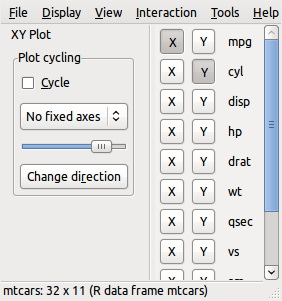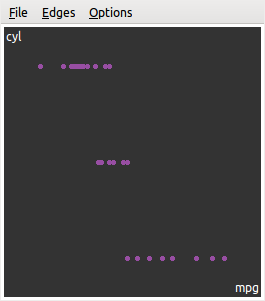图 5.16: GGobi 软件的主窗口（左）和图形窗口（右）：我们可以在主窗口中选择要画散点图的两个变量。 主窗口中有一套菜单系统，左侧是一些图形控制选项，右侧是当前画图所用的变量。下面我们简单介绍一下菜单： Display 新建图形类型：我们可以用散点图、散点图矩阵、平行坐标图、时间序列图和条形图，前四种基本上是针对连续数据的，而条形图则适合离散数据，这些图形我们在前面章节中都已经介绍过 View 视图：可以是一维图（实际上是一维密度曲线）、XY 散点图、一维巡游（Tour）、三维旋转和二维巡游等，巡游是一种很有用的视图模式，后面我们详细介绍 Interaction 交互：可以是缩放（Zoom）和平移（Pan）、选取（Brush）、识别（Identify）和移动点等；缩放可以通过鼠标滚轮实现，平移可以用鼠标点住图形往任意方向拖动，选取则可以通过一个矩形框（通常称之为“刷子”）选择图中的元素如点、条等，识别可以让我们在选取元素的时候知道所选的元素的相关信息，移动则可以让我们手工操纵图形元素的位置（用鼠标把它们拖到新的位置） Tools 工具：这里面的工具较多，我们只介绍其中一种 — 自动配色（Automatic Brushing），打开这个工具之后，我们会得到一个变量列表框，选取一个变量后，图中的元素会根据这个变量来配色，尤其是数据中有分类变量时这个功能会变得非常有用，我们可以根据一个分类变量的不同类别将图形元素标记为不同颜色，这样在散点图或者巡游视图中我们可以很方便观察各分类之间的区别 交互是 GGobi 的一个重要特征，而各种交互模式中，选取可能是最重要的一种。选取背后有一套连接逻辑，即各图形窗口实际上是被连接在一起的，当我们在其中一个图形窗口中操作时，其它图形窗口也会根据当前窗口中选取的观测相应更新，这些图形窗口也许是同一种图形，也许不是同一种图形。例如我们在散点图中选中一个点，它对应着原数据的一行观测，那么另一个窗口中平行坐标图的一条线也会被刷上，因为这条线对应着同一行观测。总之，选取和连接的逻辑就是从一幅图映射到原数据的行，再从行映射到另一幅图。 选取至少有两方面的意义： 1. 让一部分数据凸显出来，让我们观察这部分数据的特征，典型的例子如离群点 2. 让“条件分割”操作变为动态操作：我们已经不止一次提到图形中的“条件分割”，如 4.12 小节的条件分割图、 lattice 系统和 ggplot2 系统的切片功能，所有的这些分割都是对数据的静态、固定分割，例如根据一个分类变量把数据分成子集；当我们使用刷子的时候，实际上也是一种条件分割：我们可以观察随着刷子位置的有序移动（如从左到右），其它图中的图形元素的位置变化，尤其是我们用鼠标右键拖动改变刷子形状为一个狭长的矩形条时，这种条件分割的意味就更加清楚，这一点可能不太好理解，参见后面思考与练习 5.24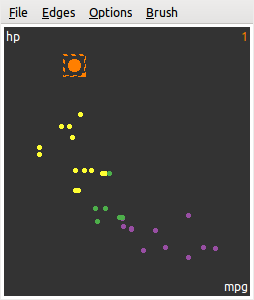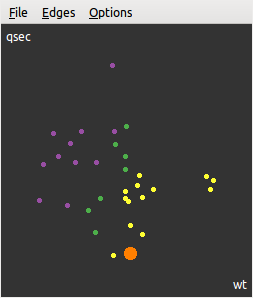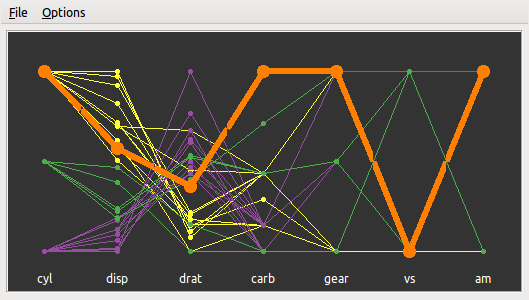图 5.17: GGobi 中选取一个观测对应所有图形窗口的更新：我们在左上角的散点图中选取一个点，右上角的散点图也相应选取了一个点，而底部的平行坐标图则选取了一条线。所有的这些选取都代表了同一行数据。 5.17 显示了一个 GGobi 刷子选取的场景，我们首先基于 mtcars 数据画了三幅图形，分别为 hpmpg 的散点图、qsecwt 的散点图以及其它七个变量的平行坐标图，其次用自动配色功能根据汽缸数量 cyl 将数据展示为三种颜色，分别对应着 4 （紫）、6 （绿）、8 （黄）个汽缸，然后我们发现第一幅散点图中似乎有个离群点 — 有一款车的马力 hp 超大且比较费油（每加仑汽油行驶英里数比较小），这款车在其它变量上有什么特征呢？通过使用刷子选取这个点（刷子颜色设置为橙色），我们观察到第二幅散点图中底部也有一个点被刷中了，这款车的重量 wt 一般，但速度超快（行驶 1/4 英里所需时间 qsec 很短），类似地，我们还可以看平行坐标图中其它指标的取值，例如它是手动档的车（am 为 1）。 交互操作在若干图形系统中都存在，且逻辑都差不多，而巡游则几乎是 GGobi 的独特特征，它让我们能够观察高维情况下数据的“形状”。在介绍巡游之前，我们必须了解一项基础知识，即投影。投影的理论很简单，它只是将高维空间映射到低维空间，这种映射通常是通过一个投影矩阵来实现的。最简单的投影例子就是影子：物体本身是三维的，但被光照投射到地面上时我们得到的是二维的形状（影子）。从数学上而言，高维的原始数据可以用矩阵表达： $X_{n\times p} = \left[\begin{array}{cccc} x_{11} & x_{12} & \cdots & x_{1p}\\ x_{21} & x_{22} & \cdots & x_{2p}\\ \vdots & \vdots & \ddots & \vdots\\ x_{n1} & x_{n2} & \cdots & x_{np} \end{array}\right]$ 其中 $$x_{ij}$$ 表示第 $$i$$ 行、第 $$j$$ 列的数据。这是一个 $$p$$ 维矩阵，如果我们要将它投影到二维平面上，则需要右乘一个 $$p\times2$$ 的投影矩阵： $A_{p\times2} = \left[\begin{array}{cc} a_{11} & a_{12}\\ a_{21} & a_{22}\\ \vdots & \vdots\\ a_{p1} & a_{p2} \end{array}\right]$ 这样我们就可以得到一个 $$n\times2$$ 的矩阵，从而可以画散点图，因为新的数据只有两列。举个最简单的例子，假设投影矩阵如下： $A = \left[\begin{array}{cc} 1 & 0\\ 0 & 1\\ 0 & 0\\ \vdots & \vdots\\ 0 & 0 \end{array}\right]$ 那么根据矩阵乘法： $XA = \left[\begin{array}{cc} x_{11} & x_{12}\\ x_{21} & x_{22}\\ \vdots & \vdots\\ x_{n1} & x_{n2} \end{array}\right]$ 不难看出，实际上这个投影就是原始数据的前两列。通常我们约束投影矩阵的列平方和为 1。 通过对投影矩阵的系数不断改变，我们可以不断得到新的散点图，这就是二维巡游的基本原理。类似的，一维巡游是将高维数据投影到一维直线上。图 5.18: GGobi 中的二维巡游模式：寻找投影将手动档和自动档的车分开。 5.18mtcars 数据的一幅二维巡游截图，图中不同颜色的点分别表示自动档（紫色）和手动档（黄色）的车。我们一共选取了 5 个变量进入巡游，如图左下角的“坐标轴”所示。这里的坐标轴显示的是一个单位圆，半径为 1，其中每个小短轴代表了各个变量的投影系数：轴的长短代表了系数的大小，方向表示投影系数的正负，每根轴都可以分解到水平方向和垂直方向上，水平方向表示对第一维的投影（即散点图的横轴），垂直方向上表示对第二维的投影（即散点图纵轴）。从图中可以看出，如果我们想把手动档和自动档的车分开，那么 mpg 这个变量不太重要，因为它的投影系数几乎为 0，wthp 对水平方向上的分离有较大贡献，qsec 对垂直方向上的分离有贡献，而 drat 变量对两个方向上都有贡献（分解到两个方向上后系数都会相对较大）。这些投影系数都可以通过菜单 Tour2D->Show Projection Vals 显示出来。 和巡游紧密相连的一个统计方法是投影寻踪（Projection Pursuit）。前面介绍的巡游若非特别设定，只是随机改变某些投影系数的值并重新画散点图，这样就生成了不断运动的点。投影寻踪顾名思义，是要寻找投影系数让我们能够发现特殊的现象，并非让巡游图随机巡游。这里的特殊现象有很多种，我们可以从 GGobi 主窗口中打开投影寻踪窗口并选择我们想要的寻踪方式，例如寻找投影使得“散点云”中的“空洞”最大，或者寻找线性判别分析（LDA）的系数，让分类变量的各类能尽量分开。图 5.18 实际上是 LDA 的结果，我们可以看到黄色的点都在图的左下角，紫色的点都在右上角。在进行巡游时，我们同样可以使用刷子选取点，有时候一些数据点在一种投影下不是离群点，但换一种投影则会变成离群点，这种情况下刷子能帮我们标记这种点，进而研究它究竟有什么特殊之处。 本节仅仅对 GGobi 做了初步介绍，关于它的更多功能，读者可以通过配套书籍 Cook and Swayne (2007) 了解，该书中有大量的实际案例，充分展现了 GGobi 在数据可视化和模型可视化方面的探索功能。 GGobi 擅长的是连续数据的动态和交互式图形，关于离散数据的交互式图形系统，Mondrian 可能是更好的选择，参见 http://www.theusrus.de/Mondrian/；另外还有一个基于 Java 的 R 包 iplots 也可以是一种选择。 本作者也一直在参与开发 GGobi 的下一代（GGobi 本身已经几乎停止开发），我们的工作基于诺基亚的 Qt，它的交互性能更好，而且 GGobi 的原开发人员也提供了从 Qt 到 R 的 API 接口，这使得我们的新软件可以利用 R 自身强大的统计计算功能，功能上可能超越现有的任何动态与交互式图形系统，关于这些开发工作，感兴趣的读者可以访问 https://github.com/ggobi ## 5.5 rgl 三维图形 rgl 是 R 的一个附加包（名字来自 R 和 OpenGL），利用它可以在 R 中绘制可交互的三维图形，用户通过鼠标的点击拖拽和滚轮滚动可以对图形进行旋转和缩放等操作。rgl 包提供了一系列的函数来绘制三维图形，这些函数分为两类，一类是 xxx3d()，如 plot3d()points3d()lines3d()persp3d() 等，另一类是 rgl.xxx()，如 rgl.lines()rgl.points()。这两类函数通常不建议混用，本节只介绍前一类。不难看出，这些函数的名字是与基础图形系统中的 plot()points() 等函数相对应的，它们的用法也有很多相似的地方，因此只要我们掌握了基础图形的绘制，rgl 包也会变得很容易学习。 ### 5.5.1 三维点线图 绘制三维点线的方法与二维时非常相像，只需使用 plot3d() 函数并提供 x 轴、y 轴和 z 轴的坐标向量即可。此外，plot3d() 函数还提供了 type 参数，用来指定图形的形式，其可能的取值有 pslh，分别表示绘制点、球体、折线和到零点的垂线。如果要在已有的图形中加上新的点或线，则可以使用 points3d()lines3d() 函数，其用法与 plot3d() 类似。 library(rgl) usage(plot3d, "default") ## plot3d(x, ...) 5.19 提供了一个简单的示例，即模拟甲烷分子（$$\mathrm{CH_{4}}$$）的构造。 x <- c(0, 0, 1, 1, 0.5) y <- c(1, 0, 1, 0, 0.5) z <- c(1, 0, 0, 1, 0.5) plot3d(x, y, z, type = "s", xlab = "", ylab = "", zlab = "", box = FALSE, axes = FALSE, radius = c(rep(0.2, 4), 0.4), col = c(rep("blue", 4), "black") ) ind <- c(5, 1, 5, 2, 5, 3, 5, 4) lines3d(x[ind], y[ind], z[ind], lwd = 2)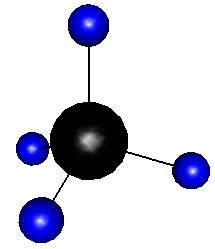图 5.19: 用 rgl 包画出来的甲烷分子立体结构：四个氢原子和一个碳原子。 ### 5.5.2 三维透视图 三维透视图对于探索二元函数的形状非常有帮助。在基础图形系统中，已经有 persp() 函数可以绘制三维透视图，但其视角是固定的，无法进行交互。rgl 包中提供了类似的函数 persp3d()，它支持对图形进行旋转和缩放，因此可以方便地观察图形的每个细节。 x <- y <- seq(1, 5, .1) m <- outer(x, y, function(a, b) beta(a, b)) persp3d(x, y, m, col = "green3", zlab = "Beta(x, y)")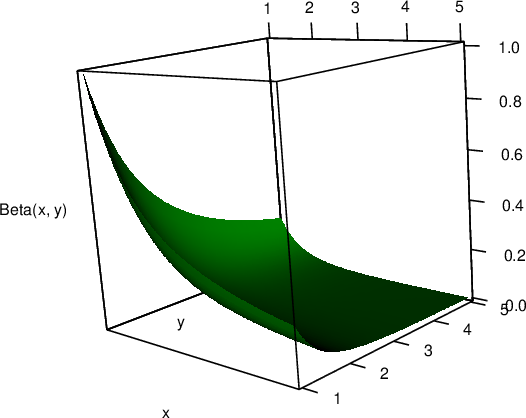图 5.20: Beta 函数的三维透视图：$$\mathrm{Beta}(x,\,y) = \Gamma(x)\Gamma(y)/\Gamma(x+y)$$ 函数中 xy 都是一个向量，z 是一个矩阵，它第 $$i$$ 行第 $$j$$ 列的元素就是函数在 x[i]y[j] 上的取值；z 矩阵一般可以通过 outer() 函数生成，但其中的 FUN 参数必须是向量化的函数。图 5.20 展示了二元函数 $$z = \mathrm{Beta}(x,\,y) = \Gamma(x)\Gamma(y)/\Gamma(x+y) = \int_{0}^{1}t^{x-1}(1-t)^{y-1}\,\mathrm{dt}$$ 的形状。persp3d() 还有一些有趣的应用，例如图 5.21 绘制出了中国及周边地区的地势图，图中可以很清楚看见青藏高原、塔里木盆地、四川盆地等我们熟悉的地区；为了更好地显示 3D 效果，高度在图中按比例调整过。 # 读入地势数据 mat <- as.matrix(read.csv(system.file("extdata", "ChinaGeoMap.csv", package = "MSG"))) x <- 20 * (1:nrow(mat)) y <- 20 * (1:ncol(mat)) z <- 0.05 * mat persp3d(x, y, z, xlab = "", ylab = "", zlab = "", col = "green", aspect = "iso", axes = FALSE, box = FALSE )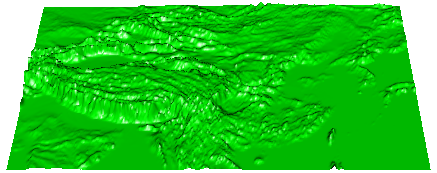图 5.21: 基于 rgl 包绘制的中国地势图 ### 5.5.3 动画和截图 在 rgl 绘图设备中，除了通过鼠标拖动来控制图形的旋转，还可以调用函数来使图形“自动播放”。感兴趣的读者可以运行 demo('flag', package = 'rgl') 来观看动画效果，其原理就是使用了 play3d() 函数； play3d() 函数的第一个参数 f 是一个控制绘图参数的函数，一般可以通过 spin3d()par3dinterp() 这两个函数生成，具体的细节可以参阅 rgl 包自带的帮助文档。 如果想将 rgl 动画保存下来，则可以使用 movie3d() 函数。下面的命令会将动画逐帧保存为 PNG 图片，存放在当前的工作目录下。 library(rgl) open3d() plot3d(cube3d(col = "green")) movie3d(spin3d(), duration = 5, convert = FALSE, dir = ".") 如果系统中安装了 ImageMagick 软件，则可以在上述命令中指定 convert = TRUE，这可以使动画保存为单个的 GIF 文件。除此之外，rgl 包还提供了 snapshot3d() 函数来保存单张的窗口截图。用户只需将三维图形调整到合适的角度，然后调用这个函数即可。5.6 小节的动画包也可以很方便抓取 rgl 图形直接生成动画。 ## 5.6 动画视频 作者的个人兴趣之一是统计学动画，这里也简要介绍一下动画的基本原理以及它与统计学理论的内在联系。animation(Xie 2013) 利用 R 基础图形系统生成了一系列统计学动画；从这个附加包的展示中，读者可以更加深刻认识到对图形元素的控制是一门具有广泛意义的艺术。 动画的基本原理很简单，即快速连续展示一幅幅静态图形，利用人眼的视觉错觉功能造成动态效果；下文中我们把一幅静态图形称为动画的一帧。在 R 中我们可以快速生成一系列的静态图片并播放出来，这样就可以构成基本的动画了。整个动画包的代码设计方案如下： library(animation) oopt <- ani.options(interval = 0.2, nmax = 10) # 设置动画选项 # 用一个循环不断创建静态图形 for (i in 1:ani.options("nmax")) { draw_plot() # 画图 ani.pause() # 停顿（用 ani.options('interval') 设置） } ani.options(oopt) # 重置动画选项 其中 ani.options() 可以用来设定一些和动画有关的选项，例如 interval 是动画中的停顿时间，nmax 是循环的次数，通常也是动画的总帧数。上面的代码大意就是用一个循环不断画图，每一次画图之后停顿一小会儿。这里的停顿是基于 R 函数 Sys.sleep() 实现的，后面我们可以看到 ani.pause() 函数的源代码。首先我们以一个具体实例“布朗运动”说明动画构建过程，参见 animation 包中的函数 brownian.motion() library(animation) brownian.motion ## function (n = 10, xlim = c(-20, 20), ylim = c(-20, 20), ...) ## { ## x = rnorm(n) ## y = rnorm(n) ## for (i in seq_len(ani.options("nmax"))) { ## dev.hold() ## plot(x, y, xlim = xlim, ylim = ylim, ...) ## text(x, y) ## x = x + rnorm(n) ## y = y + rnorm(n) ## ani.pause() ## } ## } ## <bytecode: 0x7f78ce493e00> ## <environment: namespace:animation> # 函数 ani.pause() 定义如下 ani.pause ## function (interval = ani.options("interval")) ## { ## if (dev.interactive()) { ## dev.flush() ## Sys.sleep(interval) ## } ## } ## <bytecode: 0x7f78ce4fbe00> ## <environment: namespace:animation> 布朗运动就是一些点在平面上随机游走，下一次的位置坐标是在上一次的位置坐标上加上独立同分布的正态随机数。注意这个动画中我们需要固定图的坐标范围，这样所有的点的位置才有一个固定的参照系，从而显示出“动感”。 animation 包主要有两方面的贡献，一方面是它有一套完善的动画导出工具，另一方面是它收录了大量的统计学主题相关的动画函数。下面我们分别介绍包中的导出工具和统计学主题。 gifski 将 PNG 图形合成制作 GIF 动态图形 for (i in 1:2) { pie(c(i %% 2, 6), col = c('red', 'yellow'), labels = NA) }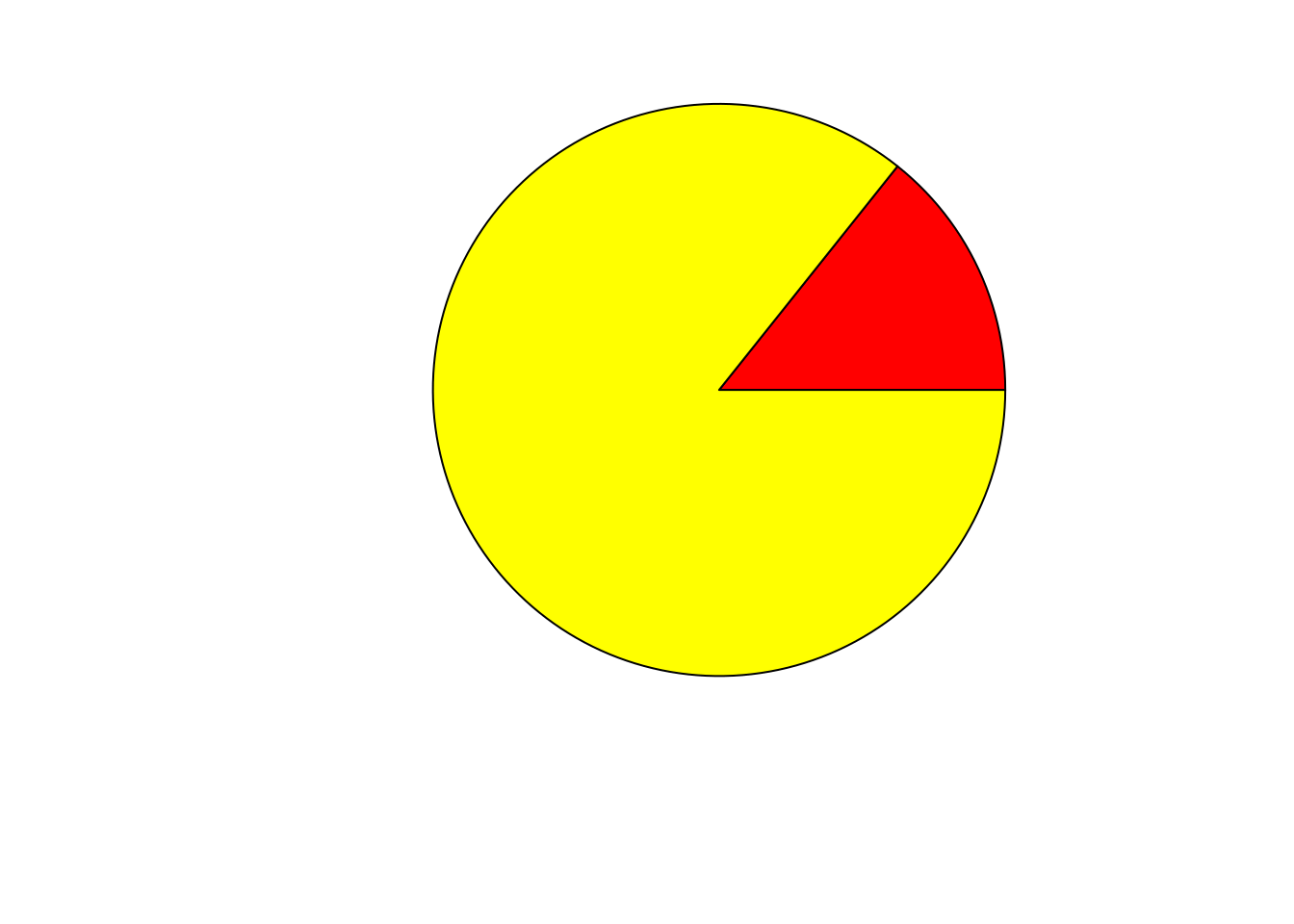上面那个例子是用 Base R 生成基本图形，下面这个例子将使用 ggplot2 生成的图形，并结合 gganimate 制作动画 https://github.com/thomasp85/gganimate library(ggplot2) library(gganimate) ggplot(mtcars, aes(factor(cyl), mpg)) + geom_boxplot() + # Here comes the gganimate code transition_states( gear, transition_length = 2, state_length = 1 ) + enter_fade() + exit_shrink() + ease_aes('sine-in-out') ### 5.6.1 动画导出工具 根据前面的描述，我们已经可以马上写一些 R 动画，但是我们会立刻遇到问题：因为 R 图形默认显示在图形窗口中，而 R 的图形窗口对双重缓冲（double buffering）的支持还不太完善，目前只是 Windows 下的图形窗口支持双重缓冲，Linux 和 Mac OS 的图形窗口都会因为不支持缓冲而导致动画很“卡”。此外，按照前面的代码方案，在 R 里面播放动画时所有的计算都得重新实时进行，这些计算可能会很耗时间。综合这两个原因，我们可能需要将 R 中的动画导出为其它可以直接观看的格式。animation 包提供了五种导出格式，对应着以下五个函数： saveHTML() HTML 格式：将所有的帧用图形设备（B.6 小节）记录为图片文件，并生成一个 HTML 页面，其中用 JavaScript 调用这些静态图片逐个显示形成动画；这个 HTML 页面的界面就像一个影音播放器，有开始/停止/前进/后退等功能，特别值得一提的是这个函数会把制作动画的 R 代码也写入 HTML 页面，这样观看动画的用户也能知道页面内的动画是如何生成的 saveLatex() PDF 格式：同样用图形设备记录所有帧并写入一个 LaTeX 文件，然后基于 LaTeX 宏包 animate 将这些帧编译为 PDF 文件中的动画，可以用 Adobe Reader 在页面内直接观看动画（其它 PDF 阅读器无法观看），实际上这里的动画也是用 JavaScript 驱动的；注意 saveLatex() 函数可以结合 Sweave 实时生成动画，这样我们可以将动画动态嵌入 LaTeX 文档 saveGIF() GIF 动画格式：GIF 是一种最常见的动画图片格式，这里我们调用的是第三方软件 ImageMagick 或者 GraphicsMagick 将 R 的图片转化为 GIF 动画，因此用户必须安装这两个软件中的一种（都是免费开源软件） saveSWF() Flash 格式：这也是网络上最常见的动画格式，这个函数可以调用第三方软件 SWFTools 将 R 图片转化为 Flash 动画 saveVideo() 影音格式：调用第三方软件 FFmpeg 将图片转化为常见的影音格式，如 AVI 和 MP4 等 这五种导出方式中，saveHTML() 是最便利的，它不需要借助任何第三方软件，而且通常我们都有网页浏览器（如 Firefox ），这样就足够观看动画了。这些函数的用法都比较类似，一般情况下我们只需要提供一段生成多幅图片的 R 代码，利用默认参数设置就足够导出动画。它们的具体用法如下： usage(saveHTML) ## saveHTML(expr, img.name = "Rplot", global.opts = "", single.opts = "", ## navigator = ani.options("nmax") <= 100 && ani.options("interval") >= 0.05, ## htmlfile = "index.html", ...) usage(saveLatex) ## saveLatex(expr, nmax, img.name = "Rplot", ani.opts, centering = TRUE, ## caption = NULL, label = NULL, pkg.opts = NULL, documentclass = "article", ## latex.filename = "animation.tex", pdflatex = "pdflatex", ## install.animate = TRUE, overwrite = TRUE, full.path = FALSE, ...) usage(saveGIF) ## saveGIF(expr, movie.name = "animation.gif", img.name = "Rplot", ## convert = "magick", cmd.fun, clean = TRUE, extra.opts = "", ...) usage(saveSWF) ## saveSWF(expr, swf.name = "animation.swf", img.name = "Rplot", swftools = NULL, ## ...) usage(saveVideo) ## saveVideo(expr, video.name = "animation.mp4", img.name = "Rplot", ## ffmpeg = ani.options("ffmpeg"), ## other.opts = if (grepl("[.]mp4$", video.name)) "-pix_fmt yuv420p", ...)

saveHTML({
ani.options(interval = 0.05, nmax = 50)
par(mar = c(4, 4, .1, 0.1), mgp = c(2, 0.7, 0))
brownian.motion(pch = 21, cex = 5, col = "red", bg = "yellow")
},
img.name = "bm-plot",
title = "Demonstration of the Brownian Motion",
description = c(
"Random walk", "on the 2D plane: for each point",
"(x, y), x = x + rnorm(1) and y = y + rnorm(1)."
), ani.height = 300, ani.width = 550
)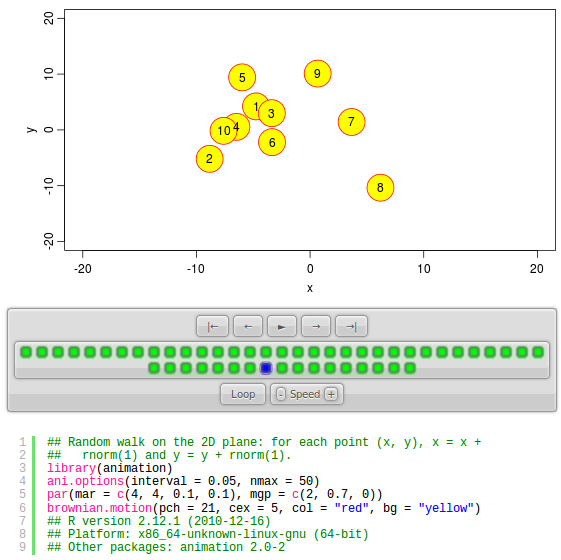### 5.6.2 统计学动画

Bootstrap 是应用最广泛的统计方法之一，它的核心就是有放回的重抽样，这个过程同随机模拟一样，也需要重复多次，我们也可以用动画展示每次重抽样的结果，animation 包中的 boot.iid() 函数就是这样的一个演示

demo("rgl_animation", package = "animation")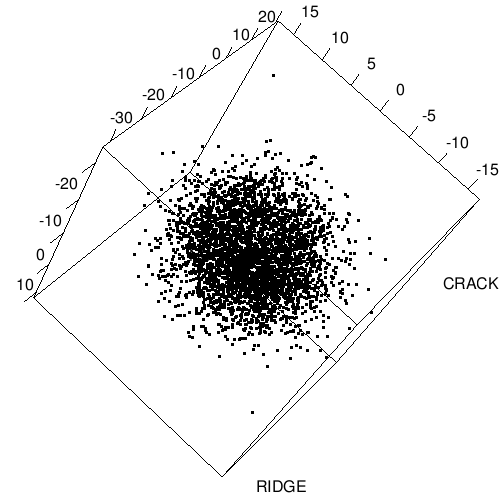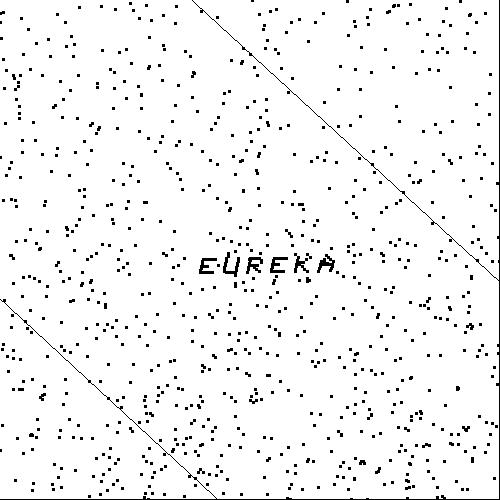## 5.7 思考与练习

1. lattice 和 ggplot2 系统都可以用两个或多个分类变量拆分数据形成数据子集并分别画图，当这些分类变量的类数特别多的时候，图形必然会变得非常拥挤，因为画布被拆分为很小的矩形块，此时我们也几乎无法从图中清楚看到数据的特征，对这种情况你有什么解决办法？动态图形系统或者动画是否有帮助？如果我们感兴趣的只是某一个特定的子集，我们是否可以通过数据变换的方式来凸显这个子集？（例如新生成一个逻辑变量来标明某些行是否属于这个子集）

2. ggplot2 默认背景为灰色的理由是否令你信服？

3. 5.5 的上图和下图看起来唯一的区别就是箱线图的方向不同，但下图至少从排版上而言略占优势，这个优势是什么？

4. ggplot2 包中的钻石数据 diamonds 中提供了钻石的长 xyz 三个变量，请用这些变量画散点图并指出这批数据可能存在的问题。

5. 对钻石数据的重量 carat 和价格 price 画散点图，其中不同的 cut 分类标记不同颜色，并观察：

1. 图中是否有异常区域？例如空白区域。克拉数的分布是否均匀？

2. 图中五种颜色的点是否清楚可辨？如果觉得某些点覆盖了另一些点，那么你是否有办法对这幅散点图进行简化？（提示：可以不画点，只画平滑曲线）

3. 对数据作对数变换是否能增强图形的可读性？

6. 在介绍 GGobi 时我们提到动态的“条件分割”，请思考当刷子在散点图中从左到右或者从下到上移动时，对数据来说，我们实际上是在选取具有何种特征的子集？如图 5.24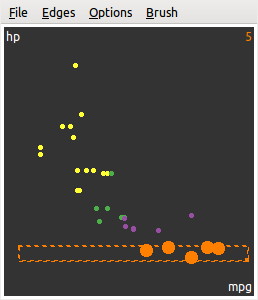图 5.24: GGobi 中用刷子实现动态条件分割：这把狭长的刷子可以从下到上移动。

7. fun 中有一个 3D 版本的“我的中国心”，效果如图 5.25；请对照 demo('ChinaHeart3D', package = 'fun') 以及代码中的源链接思考它的制作过程，并学习如何将平面图形嵌入 rgl 三维图形。图 5.25: 3D 版本的“中国心”：基于 rgl 包和求根函数制成。

8. 网络上已经有无数的关于中心极限定理（CLT）的动画展示，大意就是随着样本量增加，样本均值 $$\bar{X}_{n}$$ 的分布密度曲线逐渐变成了“钟形曲线”，而正态分布的密度曲线也是钟形曲线，所以人们就下结论说 CLT 成立。这样的结论是否可靠？animation 包中的 clt.ani() 函数也可以用来演示 CLT，但角度略有不同，它将每个样本量下的样本均值拿来做了正态性检验，并将相应的 P 值画出来，以刻画当前的这些样本均值和正态分布的拟合好坏，这样做是否让 CLT 的动画演示更加严谨？

9. 比较 animation 包中的五种导出格式：HTML、PDF、GIF、Flash 和视频，它们各自有什么优势劣势？（如：HTML 页面适合网络发布而且对播放器的要求非常低，但它包含了太多文件，不方便复制传播）

### 参考文献

Carr, Dan, Nicholas Lewin-Koh, and Martin Maechler. 2021. Hexbin: Hexagonal Binning Routines. https://github.com/edzer/hexbin.
———. 1993. Visualizing Data. Hobart Press.
Cook, Dianne, and Deborah F. Swayne. 2007. Interactive and Dynamic Graphics for Data Analysis with r and GGobi. Springer.
Lawrence, Michael, and Duncan Temple Lang. 2010. RGtk2: A Graphical User Interface Toolkit for R.” Journal of Statistical Software 37 (8): 1–52. https://www.jstatsoft.org/v37/i08/.
Sarkar, Deepayan. 2008. Lattice: Multivariate Data Visualization with r. New York, NY: Springer. http://lmdvr.r-forge.r-project.org.
Theus, Martin. 2002. “Interactive Data Visualization Using Mondrian.” Journal of Statistical Software 7 (11): 1–9. https://www.jstatsoft.org/v07/i11.
Urbanek, Simon, and Tobias Wichtrey. 2018. Iplots: iPlots - Interactive Graphics for r. https://CRAN.R-project.org/package=iplots.
Wickham, Hadley. 2016. Ggplot2: Elegant Graphics for Data Analysis. 2nd ed. Springer-Verlag New York. https://ggplot2-book.org/.
Wickham, Hadley, Duncan Temple Lang, Debby Swayne, and Michael Lawrence. 2018. rggobi: Interface Between R and GGobi. https://CRAN.R-project.org/package=rggobi.
Wilkinson, Leland. 2005. The Grammar of Graphics. 2nd ed. New York, NY: Springer. https://doi.org/10.1007/0-387-28695-0.
———. 2013. animation: An R Package for Creating Animations and Demonstrating Statistical Methods.” Journal of Statistical Software 53 (1): 1–27. https://www.jstatsoft.org/v53/i01/.
Xie, Yihui, Yixuan Qiu, and Taiyun Wei. 2020. Fun: Use r for Fun. https://github.com/yihui/fun.